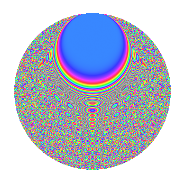# Properties

 Label 1890.2.dbLevel 1890 Weight 2 Character orbit db Rep. character $$\chi_{1890}(209,\cdot)$$ Character field $$\Q(\zeta_{18})$$ Dimension 864 Sturm bound 864

# Learn more about

## Defining parameters

 Level: $$N$$ = $$1890 = 2 \cdot 3^{3} \cdot 5 \cdot 7$$ Weight: $$k$$ = $$2$$ Character orbit: $$[\chi]$$ = 1890.db (of order $$18$$ and degree $$6$$) Character conductor: $$\operatorname{cond}(\chi)$$ = $$945$$ Character field: $$\Q(\zeta_{18})$$ Sturm bound: $$864$$

## Dimensions

The following table gives the dimensions of various subspaces of $$M_{2}(1890, [\chi])$$.

Total New Old
Modular forms 2640 864 1776
Cusp forms 2544 864 1680
Eisenstein series 96 0 96

## Trace form

 $$864q$$ $$\mathstrut +\mathstrut 12q^{9}$$ $$\mathstrut +\mathstrut O(q^{10})$$ $$864q$$ $$\mathstrut +\mathstrut 12q^{9}$$ $$\mathstrut +\mathstrut 12q^{11}$$ $$\mathstrut -\mathstrut 6q^{14}$$ $$\mathstrut -\mathstrut 12q^{15}$$ $$\mathstrut -\mathstrut 72q^{21}$$ $$\mathstrut -\mathstrut 12q^{29}$$ $$\mathstrut -\mathstrut 72q^{30}$$ $$\mathstrut +\mathstrut 54q^{35}$$ $$\mathstrut -\mathstrut 12q^{36}$$ $$\mathstrut +\mathstrut 132q^{39}$$ $$\mathstrut -\mathstrut 18q^{49}$$ $$\mathstrut -\mathstrut 36q^{50}$$ $$\mathstrut +\mathstrut 6q^{56}$$ $$\mathstrut +\mathstrut 36q^{60}$$ $$\mathstrut -\mathstrut 432q^{64}$$ $$\mathstrut +\mathstrut 72q^{65}$$ $$\mathstrut +\mathstrut 72q^{79}$$ $$\mathstrut -\mathstrut 36q^{81}$$ $$\mathstrut -\mathstrut 12q^{84}$$ $$\mathstrut +\mathstrut 120q^{95}$$ $$\mathstrut -\mathstrut 72q^{99}$$ $$\mathstrut +\mathstrut O(q^{100})$$

## Decomposition of $$S_{2}^{\mathrm{new}}(1890, [\chi])$$ into irreducible Hecke orbits

The newforms in this space have not yet been added to the LMFDB.

## Decomposition of $$S_{2}^{\mathrm{old}}(1890, [\chi])$$ into lower level spaces

$$S_{2}^{\mathrm{old}}(1890, [\chi]) \cong$$ $$S_{2}^{\mathrm{new}}(945, [\chi])$$$$^{\oplus 2}$$